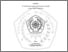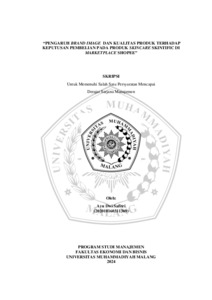## SOLUSI PERSAMAAN DIFERENSIAL LINIER HOMOGEN ORDE DUA DENGAN MENGGUNAKAN DERET PANGKAT

Sudarno, Sudarno (2008) SOLUSI PERSAMAAN DIFERENSIAL LINIER HOMOGEN ORDE DUA DENGAN MENGGUNAKAN DERET PANGKAT. Other thesis, University of Muhammadiyah Malang.Preview
Text
SOLUSI_PERSAMAAN_DIFERENSIAL_LINIER_HOMOGEN_ORDE_DUA_DENGAN_MENGGUNAKAN_DERET_PANGKAT.pdf

## Abstract

Target of study is to know common public solution of equation of homogeneous linear differencial order two at ordinary point and point of singular regular. Method which is used in this writing literature study method that is studying from various source of like journal and books. Result of study and solution of the following: common public Solution of differencial equation in ordinary point will be taken example by rank series in form of and searched by first generation and both second. Result of its generation substitution will to differencial equation knew, to yield formula of rekursif, from formula rekursif got by common public solution of differencial equation which in the form of function of polynomial.While solution differencial equation in point of singular regular shall be as follows: ( 1) solution common public of differencial equation will be taken example in form of rank series and searched by first generation and both. Its generation substitution to differencial equation knew to yield formula of rekursif, from formula of rekursif got by first solution which in form of. ( 2) both second solution depend on form equation of his characteristic, if , than with , if , than with and if (positive integer), than with amount of from first solution and second will be got by common public solution of differencial equation which in the form of function of polynomial.

Item Type: Thesis (Other) L Education > L Education (General) Faculty of Teacher Training and Education > Department of Mathematics Education (84202) Rayi Tegar Pamungkas 13 Jun 2012 03:04 13 Jun 2012 03:04 http://eprints.umm.ac.id/id/eprint/7492View Item